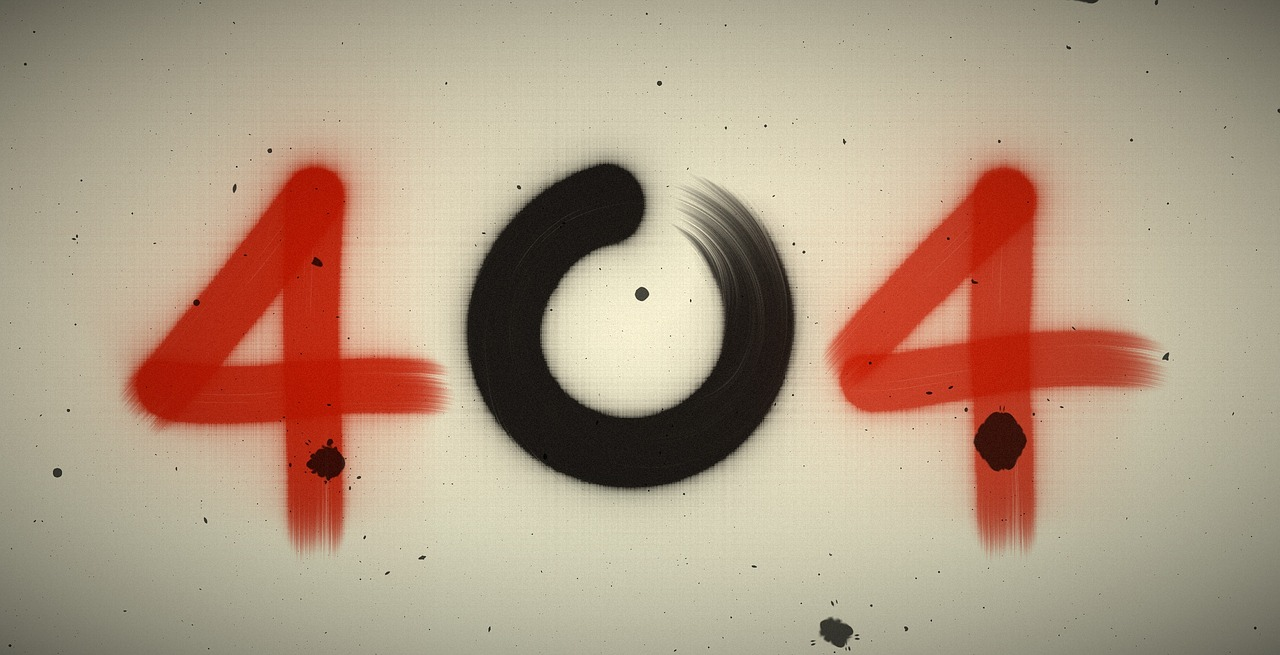## Post per Page

In physics, there are two main methods to model the universe. The first one or the known one is the classical way. Classical models such as Newton’s laws of motion and Einstein’s theory of relativity suggest that the characteristics of an object such as its position and motion are absolute. There are practical limits to how accurately we can measure an object’s path through space and time, but that’s on us. Nature knows their motion with infinite precision.

Quantum models such as atomic physics assume that objects are governed by interactions. These interactions are probabilistic and indefinite. Even if we constrain an interaction to limited outcomes, we can never know the motion of an object with infinite precision, because nature doesn’t allow it.

These two theoretical worlds, the definite classical and indefinite quantum, each work extremely well. The classical for large, massive objects such as baseballs and planets, and the quantum for small, light objects such as atoms and molecules. But both of these approaches break down when we try to study massive but small things such as the interiors of black holes, or the observable universe in the earliest moments of the big bang. For that has all the properties of general relativity with all the properties of quantum theory. This theory is sometimes referred to as quantum gravity, but right now we don’t know it would work.

How many theories are related. Credit: B. Jankuloski

It isn't easy to study this theory because we don’t have any experiments to prove it directly. But a new research  proposes an experiment that could provide us a glimpse of how quantum gravity might performs its action.

The key is to have an entity or object that is quantum in nature, but big enough that classical gravity has an effect. To do this the physicists proposes using a super-cooled state of matter known as Bose-Einstein condensate. This occurs when certain groups of atoms are cooled so much that they effectively blur together in a single quantum state. If billions of atoms were cooled to a Bose-Einstein condensate, they would form a single quantum object with a mass roughly equal to that of a virus. Tiny, but massive enough for the effects of gravity to be studied.

The team proposes making such a condensate, then suspending it magnetically so that only gravity can interact with it. In their work, they show that if gravity works on a quantum level, then the shape of the condensate will shift slightly from its “weightless” Gaussian shape. If gravity only interacts on a classical level, then the condensate will remain Gaussian.

This approach could be done with our current technology. Unlike other proposed studies, this experiment would only rely on a basic property of quantum systems rather than more complex interactions such as entanglement. If the experiment can be performed, it could give us the first real look at the fundamental nature of quantum gravity.

Reference: Richard Howl, et al. “Non-Gaussianity as a Signature of a Quantum Theory of Gravity.” PRX Quantum 2.1 (2021): 010325.